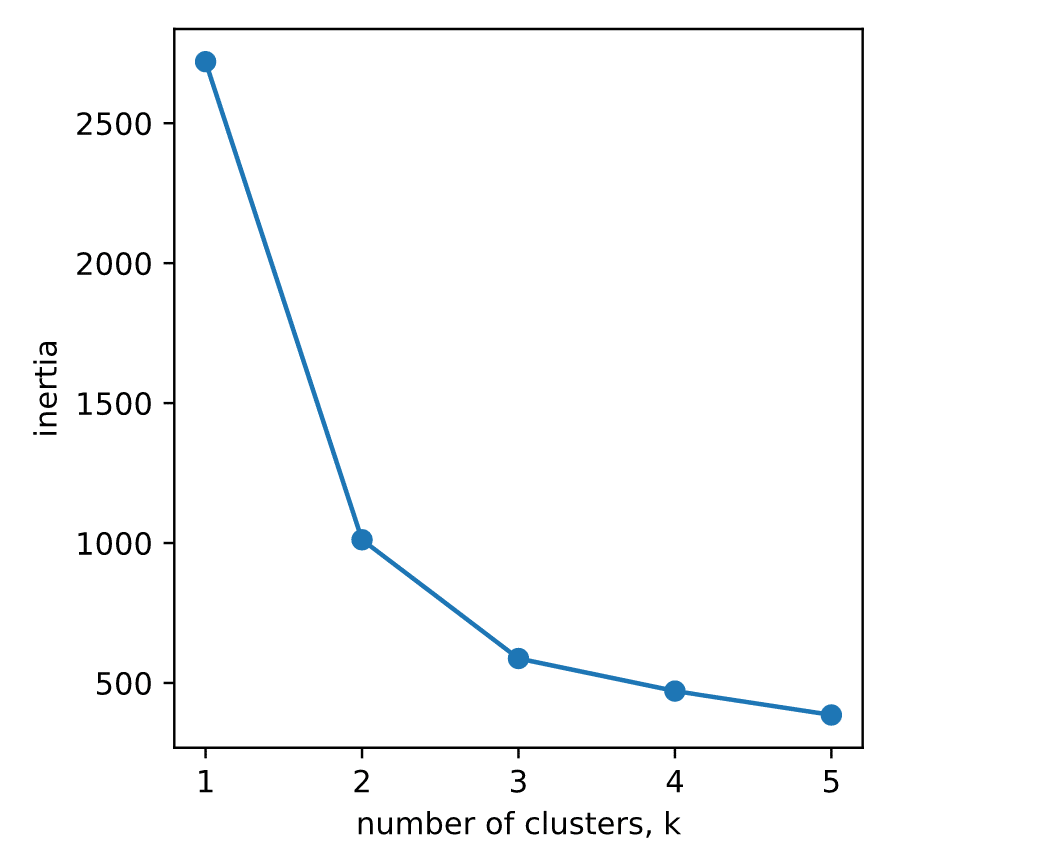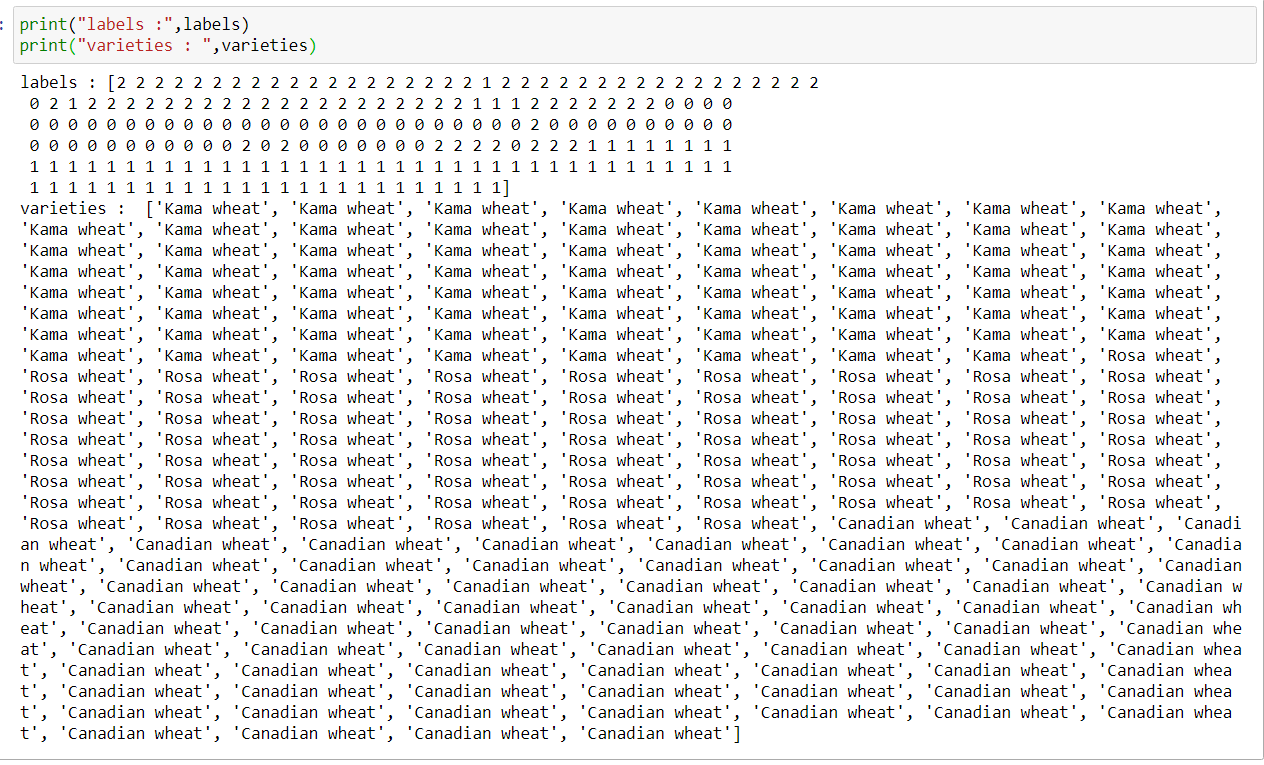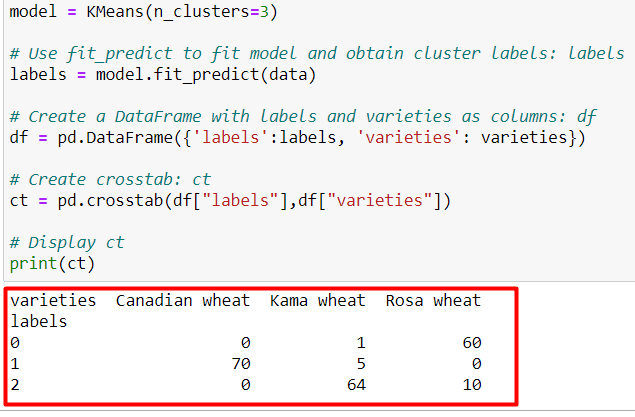# Evaluating a Clustering | Python Unsupervised Learning -2

Hi, In this article, we continue where we left off from the previous topic. If you haven’t read the previous article, you can find it here.

Python Unsupervised Learning -1

# Evaluating a Clustering

In the previous article we used k-means to cluster the sample dataset into the three cluster. But how can we evulate the quality of this clustering?

Let’s consider the iris data set as an example.

A direct approach is to compare the clusters with the iris species  You’ll learn about this first, before considering the problem of  how to measure the quality of a clustering in a way that doesn’t require our samples to come pre-grouped into species

This measure of quality can then be used to make an informed choice about the number of clusters look for.

## Cross tabulation with pandas

• Clusters vs species is a “cross-tabulation”
• Use the pandas library

Cross tabulations like these provide great insights into which sort of samples are in which cluster.

But in most dataset the samples are not labelled by species.

# Measuring clustering quality

We need a way to measure the quality of a clustering that uses only the clusters and the samples themselves.

• Using only samples and their cluster labels
• A good clustering has tight cluster
• Samples in each cluster bunched together

### Inertia measures clustering quality

• Measures how spreed out the clusters are (lower is better)
• Distance from each sample to centroid of its cluster
• Afret fit() , available as attribute inertia_
• k-means attempts to minimize the inertia when choosing clusters

### How many cluster to choose?

• A good clustering has tight clusters (so low inertia)
• …. but not too many clusters
• Choose an “elbow” in the inertia plot
• Where inertia begins to decrease more slowly

Let’s proceed with the example now.

```import matplotlib.pyplot as plt
from sklearn import datasets
from sklearn.cluster import KMeans
import pandas as pd
import numpy as np

ks = range(1, 6)
inertias = []

for k in ks:

model = KMeans(n_clusters=k)

# Fit model to samples
model.fit(data)

# Append the inertia to the list of inertias
inertias.append(model.inertia_)

# Plot ks vs inertias
plt.plot(ks, inertias, '-o')
plt.xlabel('number of clusters, k')
plt.ylabel('inertia')
plt.xticks(ks)
plt.show()```The inertia decreases very slowly from 3 clusters to 4, so it looks like 3 clusters would be a good choice for this data.Note: labels and varieties variables are as in the picture

```model = KMeans(n_clusters=3)

# Use fit_predict to fit model and obtain cluster labels: labels
labels = model.fit_predict(data)

# Create a DataFrame with labels and varieties as columns: df
df = pd.DataFrame({'labels':labels, 'varieties': varieties})

# Create crosstab: ct
ct = pd.crosstab(df["labels"],df["varieties"])

# Display ct
print(ct)```The cross-tabulation shows that the 3 varieties of grain separate really well into 3 clusters. But depending on the type of data you are working with, the clustering may not always be this good.

Is there anything you can do in such situations to improve your clustering? You’ll find out in the next tutorial!